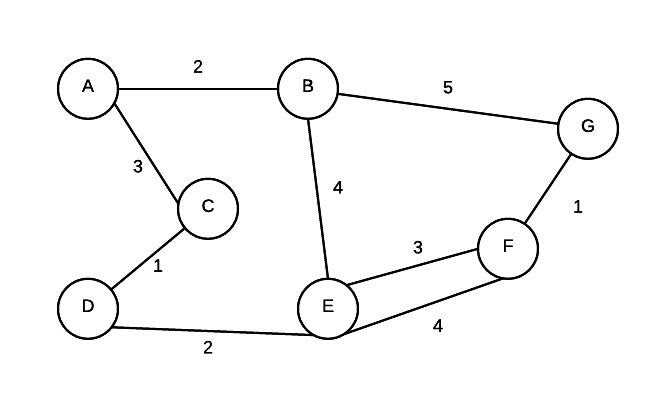# Graphs

A graph is made up of a set of vertices and edges that form connections between vertices. If the edges are directed, the graph is sometimes called a digraph. Graphs can be used to model data where we are interested in connections and relationships between data.## Definitions

• adjacent - Given two nodes A and B. B is adjacent to A if there is a connection from A to B. In a digraph if B is adjacent to A, it doesn't mean that A is automatically adjacent to B.
• edge weight/edge cost - a value associated with a connection between two nodes
• path - a ordered sequence of vertices where a connection must exist between consecutive pairs in the sequence.
• simplepath - every vertex in path is distinct
• pathlength number of edges in a path
• cycle - a path where the starting and ending node is the same
• strongly connected - If there exists some path from every vertex to every other vertex, the graph is strongly connected.
• weakly connect - if we take away the direction of the edges and there exists a path from every node to every other node, the digraph is weakly connected.